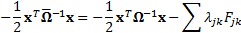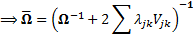/

### Calibrating probability distributions used for risk measurement purposes to market-implied data: 2. Multi-instrument calibration – Section Analysis

Next Page

2.3          A multivariate normal distribution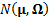with mean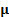(a vector of random variables) and covariance matrix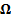has a probability density function,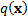as follows, whereis the number of entries in the vectorand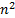the number of entries in):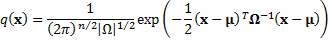2.4          We note that:

(a)    Any-dimensional multivariate normal distribution has a probability density function expressible as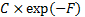where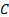is some suitable constant and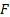is a positive definite symmetric quadratic form (with possibly non-zero drift) indifferent variables, and vice versa.

(b)   Applying analytical weighted Monte Carlo (using relative entropy) to the sort of calibration problem referred to above will therefore return (unless the calibration problem is ill-posed) a calibrated probability distribution which also has multivariate normal form. This is because the problem can be restated using Lagrange multipliers to one that involves minimising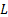defined as follows, where the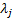refer to whatever calibrations there are on the means and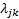to those on covariance terms (in general there will be fewer thanof the):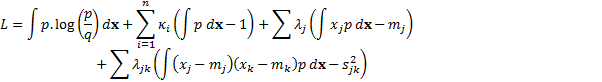The solution to this minimisation problem is given by the following: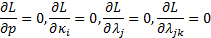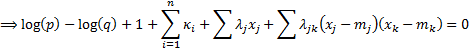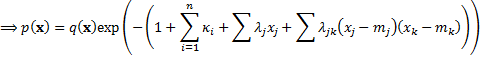subject to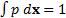(i.e. that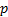is a probability distribution) and other constraints derived directly from calibration requirements, e.g. that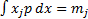etc.

Thus ifis expressible asas above, then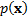will be too, just for a different.

(c)    Applying the principle of no arbitrage we may therefore expectto have zero mean (more precisely for each element ofto be the same, which without loss of generality we may take as zero if we are focusing on relative returns) and therefore to have the form: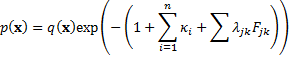where the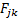are symmetric zero-drift quadratic forms (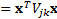, say) corresponding to each of the implied volatilities/implied correlations to which we wish to calibrate.

(d)   The calibrated distribution will therefore be multivariate normal with zero mean and probability distribution as follows, for suitably chosenthat reproduce for the calibrations the relevant market implied variances or covariances (where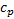is some constant the value of which ensures that):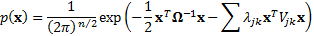(e)   Thus the calibrated probability distribution will be characterised by a covariance matrix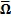as follows: